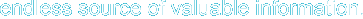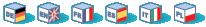# Introduction

These functions allow you to interact with and manipulate arrays in various ways. Arrays are essential for storing, managing, and operating on sets of variables.

Simple and multi-dimensional arrays are supported, and may be either user created or created by another function. There are specific database handling functions for populating arrays from database queries, and several functions return arrays.

Please see the Arrays section of the manual for a detailed explanation of how arrays are implemented and used in PHP. See also Array operators for other ways how to manipulate the arrays.

# Requirements

No external libraries are needed to build this extension.

# Installation

There is no installation needed to use these functions; they are part of the PHP core.

# Runtime Configuration

This extension has no configuration directives defined in php.ini.

# Resource Types

This extension has no resource types defined.

# Predefined Constants

The constants below are always available as part of the PHP core.

CASE_LOWER (integer)

CASE_LOWER is used with array_change_key_case() and is used to convert array keys to lower case. This is also the default case for array_change_key_case().

CASE_UPPER (integer)

CASE_UPPER is used with array_change_key_case() and is used to convert array keys to upper case.

Sorting order flags:

SORT_ASC (integer)

SORT_ASC is used with array_multisort() to sort in ascending order.

SORT_DESC (integer)

SORT_DESC is used with array_multisort() to sort in descending order.

Sorting type flags: used by various sort functions

SORT_REGULAR (integer)

SORT_REGULAR is used to compare items normally.

SORT_NUMERIC (integer)

SORT_NUMERIC is used to compare items numerically.

SORT_STRING (integer)

SORT_STRING is used to compare items as strings.

SORT_LOCALE_STRING (integer)

SORT_LOCALE_STRING is used to compare items as strings, based on the current locale. Added in PHP 4.4.0 and 5.0.2.

COUNT_NORMAL (integer)

COUNT_RECURSIVE (integer)

EXTR_OVERWRITE (integer)

EXTR_SKIP (integer)

EXTR_PREFIX_SAME (integer)

EXTR_PREFIX_ALL (integer)

EXTR_PREFIX_INVALID (integer)

EXTR_PREFIX_IF_EXISTS (integer)

EXTR_IF_EXISTS (integer)

EXTR_REFS (integer)

array_change_key_case -- Returns an array with all string keys lowercased or uppercased
array_chunk -- Split an array into chunks
array_combine --  Creates an array by using one array for keys and another for its values
array_count_values -- Counts all the values of an array
array_diff_assoc -- Computes the difference of arrays with additional index check
array_diff_key -- Computes the difference of arrays using keys for comparison
array_diff_uassoc --  Computes the difference of arrays with additional index check which is performed by a user supplied callback function
array_diff_ukey -- Computes the difference of arrays using a callback function on the keys for comparison
array_diff -- Computes the difference of arrays
array_fill -- Fill an array with values
array_filter --  Filters elements of an array using a callback function
array_flip -- Exchanges all keys with their associated values in an array
array_intersect_assoc -- Computes the intersection of arrays with additional index check
array_intersect_key -- Computes the intersection of arrays using keys for comparison
array_intersect_uassoc -- Computes the intersection of arrays with additional index check, compares indexes by a callback function
array_intersect_ukey -- Computes the intersection of arrays using a callback function on the keys for comparison
array_intersect -- Computes the intersection of arrays
array_key_exists -- Checks if the given key or index exists in the array
array_keys -- Return all the keys of an array
array_map --  Applies the callback to the elements of the given arrays
array_merge_recursive -- Merge two or more arrays recursively
array_merge -- Merge one or more arrays
array_multisort -- Sort multiple or multi-dimensional arrays
array_pop -- Pop the element off the end of array
array_product --  Calculate the product of values in an array
array_push --  Push one or more elements onto the end of array
array_rand --  Pick one or more random entries out of an array
array_reduce --  Iteratively reduce the array to a single value using a callback function
array_reverse --  Return an array with elements in reverse order
array_search --  Searches the array for a given value and returns the corresponding key if successful
array_shift --  Shift an element off the beginning of array
array_slice -- Extract a slice of the array
array_splice --  Remove a portion of the array and replace it with something else
array_sum --  Calculate the sum of values in an array
array_udiff_assoc -- Computes the difference of arrays with additional index check, compares data by a callback function
array_udiff_uassoc -- Computes the difference of arrays with additional index check, compares data and indexes by a callback function
array_udiff -- Computes the difference of arrays by using a callback function for data comparison
array_uintersect_assoc -- Computes the intersection of arrays with additional index check, compares data by a callback function
array_uintersect_uassoc -- Computes the intersection of arrays with additional index check, compares data and indexes by a callback functions
array_uintersect -- Computes the intersection of arrays, compares data by a callback function
array_unique -- Removes duplicate values from an array
array_unshift --  Prepend one or more elements to the beginning of an array
array_values -- Return all the values of an array
array_walk_recursive --  Apply a user function recursively to every member of an array
array_walk --  Apply a user function to every member of an array
array --  Create an array
arsort --  Sort an array in reverse order and maintain index association
asort -- Sort an array and maintain index association
compact --  Create array containing variables and their values
count -- Count elements in an array, or properties in an object
current -- Return the current element in an array
each --  Return the current key and value pair from an array and advance the array cursor
end --  Set the internal pointer of an array to its last element
extract --  Import variables into the current symbol table from an array
in_array -- Checks if a value exists in an array
key -- Fetch a key from an associative array
krsort -- Sort an array by key in reverse order
ksort -- Sort an array by key
list --  Assign variables as if they were an array
natcasesort --  Sort an array using a case insensitive "natural order" algorithm
natsort --  Sort an array using a "natural order" algorithm
next --  Advance the internal array pointer of an array
pos -- Alias of current()
prev -- Rewind the internal array pointer
range --  Create an array containing a range of elements
reset --  Set the internal pointer of an array to its first element
rsort -- Sort an array in reverse order
shuffle -- Shuffle an array
sizeof -- Alias of count()
sort -- Sort an array
uasort --  Sort an array with a user-defined comparison function and maintain index association
uksort --  Sort an array by keys using a user-defined comparison function
usort --  Sort an array by values using a user-defined comparison function

Hosting by: Hurra Communications Ltd.
Generated: 2007-01-26 18:00:22